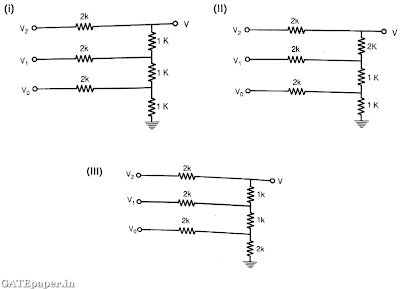### GATE 1990 Video Solutions on Digital Circuits

1. The number of Boolean functions that can be generated by n variables is equal to :2. The minimal function that can detect a “divisible by 3” 8421 BCD code digit (representation is D8D4D2D1) is given by3. A 4 bit modulo-16 ripple counter uses JK flip-flops. If the propagation delay of each flip-flop is 50 nsec, the maximum clock frequency that can be used is equal to ……
a. 20 MHz
b. 10 MHz
c. 5 MHz
d. 4 MHz

4. Which of the following resistance networks can be used as 3 bit R-2R ladder DAC? Assume Vo corresponds to LSB.a. Both (i) and (ii)
b. Both (i) and (iii)
c. Only (iii)
d. Only (ii)